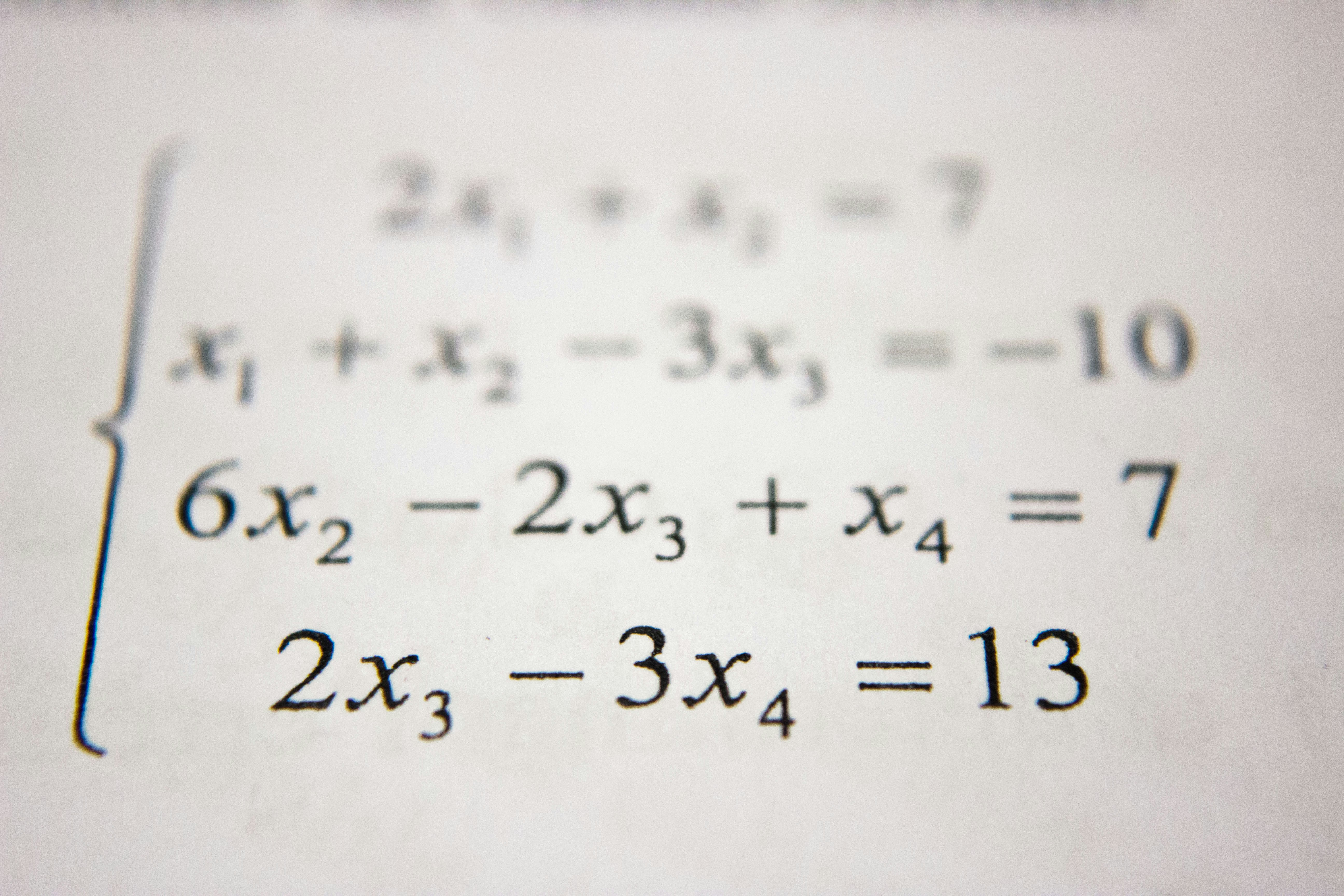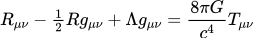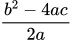LaTeX Math Reference
Share
Explore#LaTeX Math Reference

By far the most popular way to typeset formulas in books and on the web, LaTeX can also be used right here in Coda to bring beautiful mathematical expressions into your online docs.Einstein field equations.

This Coda doc is inspired by the excellent LaTeX references available online, including:

Getting started
Using LaTeX in Coda is easy: simply install the and then hit the = key to insert a new formula:

=LaTeX::Math("\frac{b^2-4ac}{2a}")You can also use the Latex→Math column format in tables to render LaTeX expressions directly. Combine that with Coda's formula language for some cool results!